Calculus Volume 3

# 2.1Vectors in the Plane

Calculus Volume 32.1 Vectors in the Plane

### Learning Objectives

• 2.1.1 Describe a plane vector, using correct notation.
• 2.1.2 Perform basic vector operations (scalar multiplication, addition, subtraction).
• 2.1.3 Express a vector in component form.
• 2.1.4 Explain the formula for the magnitude of a vector.
• 2.1.5 Express a vector in terms of unit vectors.
• 2.1.6 Give two examples of vector quantities.

When describing the movement of an airplane in flight, it is important to communicate two pieces of information: the direction in which the plane is traveling and the plane’s speed. When measuring a force, such as the thrust of the plane’s engines, it is important to describe not only the strength of that force, but also the direction in which it is applied. Some quantities, such as velocity or force, are defined in terms of both size (also called magnitude) and direction. A quantity that has magnitude and direction is called a vector. In this text, we denote vectors by boldface letters, such as v.

### Definition

A vector is a quantity that has both magnitude and direction.

### Vector Representation

A vector in a plane is represented by a directed line segment (an arrow). The endpoints of the segment are called the initial point and the terminal point of the vector. An arrow from the initial point to the terminal point indicates the direction of the vector. The length of the line segment represents its magnitude. We use the notation $‖v‖‖v‖$ to denote the magnitude of the vector $v.v.$ A vector with an initial point and terminal point that are the same is called the zero vector, denoted $0.0.$ The zero vector is the only vector without a direction, and by convention can be considered to have any direction convenient to the problem at hand.

Vectors with the same magnitude and direction are called equivalent vectors. We treat equivalent vectors as equal, even if they have different initial points. Thus, if $vv$ and $ww$ are equivalent, we write

$v=w.v=w.$

### Definition

Vectors are said to be equivalent vectors if they have the same magnitude and direction.

The arrows in Figure 2.2(b) are equivalent. Each arrow has the same length and direction. A closely related concept is the idea of parallel vectors. Two vectors are said to be parallel if they have the same or opposite directions. We explore this idea in more detail later in the chapter. A vector is defined by its magnitude and direction, regardless of where its initial point is located.

Figure 2.2 (a) A vector is represented by a directed line segment from its initial point to its terminal point. (b) Vectors $v1v1$ through $v5v5$ are equivalent.

The use of boldface, lowercase letters to name vectors is a common representation in print, but there are alternative notations. When writing the name of a vector by hand, for example, it is easier to sketch an arrow over the variable than to simulate boldface type: $v→.v→.$ When a vector has initial point $PP$ and terminal point $Q,Q,$ the notation $PQ→PQ→$ is useful because it indicates the direction and location of the vector.

### Example 2.1

#### Sketching Vectors

Sketch a vector in the plane from initial point $P(1,1)P(1,1)$ to terminal point $Q(8,5).Q(8,5).$

### Checkpoint2.1

Sketch the vector $ST→ST→$ where $SS$ is point $(3,−1)(3,−1)$ and $TT$ is point $(−2,3).(−2,3).$

### Combining Vectors

Vectors have many real-life applications, including situations involving force or velocity. For example, consider the forces acting on a boat crossing a river. The boat’s motor generates a force in one direction, and the current of the river generates a force in another direction. Both forces are vectors. We must take both the magnitude and direction of each force into account if we want to know where the boat will go.

A second example that involves vectors is a quarterback throwing a football. The quarterback does not throw the ball parallel to the ground; instead, he aims up into the air. The velocity of his throw can be represented by a vector. If we know how hard he throws the ball (magnitude—in this case, speed), and the angle (direction), we can tell how far the ball will travel down the field.

A real number is often called a scalar in mathematics and physics. Unlike vectors, scalars are generally considered to have a magnitude only, but no direction. Multiplying a vector by a scalar changes the vector’s magnitude. This is called scalar multiplication. Note that changing the magnitude of a vector does not indicate a change in its direction. For example, wind blowing from north to south might increase or decrease in speed while maintaining its direction from north to south.

### Definition

The product $kvkv$ of a vector v and a scalar k is a vector with a magnitude that is $|k||k|$ times the magnitude of $v,v,$ and with a direction that is the same as the direction of $vv$ if $k>0,k>0,$ and opposite the direction of $vv$ if $k<0.k<0.$ This is called scalar multiplication. If $k=0k=0$ or $v=0,v=0,$ then $kv=0.kv=0.$

As you might expect, if $k=−1,k=−1,$ we denote the product $kvkv$ as

$kv=(−1)v=−v.kv=(−1)v=−v.$

Note that $−v−v$ has the same magnitude as $v,v,$ but has the opposite direction (Figure 2.4).

Figure 2.4 (a) The original vector v has length n units. (b) The length of $2v2v$ equals $2n2n$ units. (c) The length of $v/2v/2$ is $n/2n/2$ units. (d) The vectors $vv$ and $−v−v$ have the same length but opposite directions.

Another operation we can perform on vectors is to add them together in vector addition, but because each vector may have its own direction, the process is different from adding two numbers. The most common graphical method for adding two vectors is to place the initial point of the second vector at the terminal point of the first, as in Figure 2.5(a). To see why this makes sense, suppose, for example, that both vectors represent displacement. If an object moves first from the initial point to the terminal point of vector $v,v,$ then from the initial point to the terminal point of vector $w,w,$ the overall displacement is the same as if the object had made just one movement from the initial point to the terminal point of the vector $v+w.v+w.$ For obvious reasons, this approach is called the triangle method. Notice that if we had switched the order, so that $ww$ was our first vector and v was our second vector, we would have ended up in the same place. (Again, see Figure 2.5(a).) Thus, $v+w=w+v.v+w=w+v.$

A second method for adding vectors is called the parallelogram method. With this method, we place the two vectors so they have the same initial point, and then we draw a parallelogram with the vectors as two adjacent sides, as in Figure 2.5(b). The length of the diagonal of the parallelogram is the sum. Comparing Figure 2.5(b) and Figure 2.5(a), we can see that we get the same answer using either method. The vector $v+wv+w$ is called the vector sum.

### Definition

The sum of two vectors $vv$ and $ww$ can be constructed graphically by placing the initial point of $ww$ at the terminal point of $v.v.$ Then, the vector sum, $v+w,v+w,$ is the vector with an initial point that coincides with the initial point of $vv$ and has a terminal point that coincides with the terminal point of $w.w.$ This operation is known as vector addition.

Figure 2.5 (a) When adding vectors by the triangle method, the initial point of $ww$ is the terminal point of $v.v.$ (b) When adding vectors by the parallelogram method, the vectors $vv$ and $ww$ have the same initial point.

It is also appropriate here to discuss vector subtraction. We define $v−wv−w$ as $v+(−w)=v+(−1)w.v+(−w)=v+(−1)w.$ The vector $v−wv−w$ is called the vector difference. Graphically, the vector $v−wv−w$ is depicted by drawing a vector from the terminal point of $ww$ to the terminal point of $vv$ (Figure 2.6).

Figure 2.6 (a) The vector difference $v−wv−w$ is depicted by drawing a vector from the terminal point of $ww$ to the terminal point of $v.v.$ (b) The vector $v−wv−w$ is equivalent to the vector $v+(−w).v+(−w).$

In Figure 2.5(a), the initial point of $v+wv+w$ is the initial point of $v.v.$ The terminal point of $v+wv+w$ is the terminal point of $w.w.$ These three vectors form the sides of a triangle. It follows that the length of any one side is less than the sum of the lengths of the remaining sides. So we have

$‖v+w‖≤‖v‖+‖w‖.‖v+w‖≤‖v‖+‖w‖.$

This is known more generally as the triangle inequality. There is one case, however, when the resultant vector $u+vu+v$ has the same magnitude as the sum of the magnitudes of $uu$ and $v.v.$ This happens only when $uu$ and $vv$ have the same direction.

### Example 2.2

#### Combining Vectors

Given the vectors $vv$ and $ww$ shown in Figure 2.7, sketch the vectors

1. $3w3w$
2. $v+wv+w$
3. $2v−w2v−w$
Figure 2.7 Vectors $vv$ and $ww$ lie in the same plane.

### Checkpoint2.2

Using vectors $vv$ and $ww$ from Example 2.2, sketch the vector $2w−v.2w−v.$

### Vector Components

Working with vectors in a plane is easier when we are working in a coordinate system. When the initial points and terminal points of vectors are given in Cartesian coordinates, computations become straightforward.

### Example 2.3

#### Comparing Vectors

Are $vv$ and $ww$ equivalent vectors?

1. $vv$ has initial point $(3,2)(3,2)$ and terminal point $(7,2)(7,2)$
$ww$ has initial point $(1,−4)(1,−4)$ and terminal point $(1,0)(1,0)$
2. $vv$ has initial point $(0,0)(0,0)$ and terminal point $(1,1)(1,1)$
$ww$ has initial point $(−2,2)(−2,2)$ and terminal point $(−1,3)(−1,3)$

### Checkpoint2.3

Which of the following vectors are equivalent?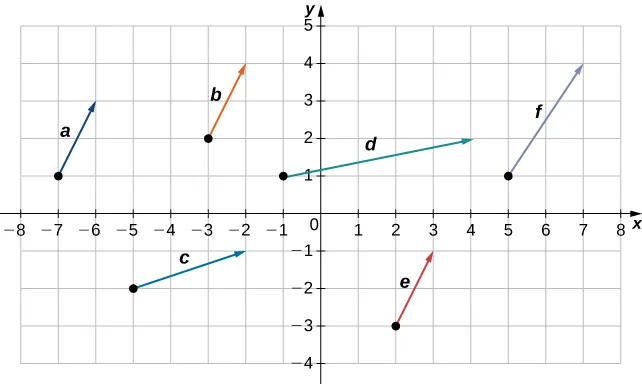We have seen how to plot a vector when we are given an initial point and a terminal point. However, because a vector can be placed anywhere in a plane, it may be easier to perform calculations with a vector when its initial point coincides with the origin. We call a vector with its initial point at the origin a standard-position vector. Because the initial point of any vector in standard position is known to be $(0,0),(0,0),$ we can describe the vector by looking at the coordinates of its terminal point. Thus, if vector v has its initial point at the origin and its terminal point at $(x,y),(x,y),$ we write the vector in component form as

$v=〈x,y〉.v=〈x,y〉.$

When a vector is written in component form like this, the scalars x and y are called the components of $v.v.$

### Definition

The vector with initial point $(0,0)(0,0)$ and terminal point $(x,y)(x,y)$ can be written in component form as

$v=〈x,y〉.v=〈x,y〉.$

The scalars $xx$ and $yy$ are called the components of $v.v.$

Recall that vectors are named with lowercase letters in bold type or by drawing an arrow over their name. We have also learned that we can name a vector by its component form, with the coordinates of its terminal point in angle brackets. However, when writing the component form of a vector, it is important to distinguish between $〈x,y〉〈x,y〉$ and $(x,y).(x,y).$ The first ordered pair uses angle brackets to describe a vector, whereas the second uses parentheses to describe a point in a plane. The initial point of $〈x,y〉〈x,y〉$ is $(0,0);(0,0);$ the terminal point of $〈x,y〉〈x,y〉$ is $(x,y).(x,y).$

When we have a vector not already in standard position, we can determine its component form in one of two ways. We can use a geometric approach, in which we sketch the vector in the coordinate plane, and then sketch an equivalent standard-position vector. Alternatively, we can find it algebraically, using the coordinates of the initial point and the terminal point. To find it algebraically, we subtract the x-coordinate of the initial point from the x-coordinate of the terminal point to get the x component, and we subtract the y-coordinate of the initial point from the y-coordinate of the terminal point to get the y component.

### Rule: Component Form of a Vector

Let v be a vector with initial point $(xi,yi)(xi,yi)$ and terminal point $(xt,yt).(xt,yt).$ Then we can express v in component form as $v=〈xt−xi,yt−yi〉.v=〈xt−xi,yt−yi〉.$

### Example 2.4

#### Expressing Vectors in Component Form

Express vector $vv$ with initial point $(−3,4)(−3,4)$ and terminal point $(1,2)(1,2)$ in component form.

### Checkpoint2.4

Vector $ww$ has initial point $(−4,−5)(−4,−5)$ and terminal point $(−1,2).(−1,2).$ Express $ww$ in component form.

To find the magnitude of a vector, we calculate the distance between its initial point and its terminal point. The magnitude of vector $v=〈x,y〉v=〈x,y〉$ is denoted $‖v‖,‖v‖,$ or $|v|,|v|,$ and can be computed using the formula

$‖v‖=x2+y2.‖v‖=x2+y2.$

Note that because this vector is written in component form, it is equivalent to a vector in standard position, with its initial point at the origin and terminal point $(x,y).(x,y).$ Thus, it suffices to calculate the magnitude of the vector in standard position. Using the distance formula to calculate the distance between initial point $(0,0)(0,0)$ and terminal point $(x,y),(x,y),$ we have

$‖v‖=(x−0)2+(y−0)2=x2+y2.‖v‖=(x−0)2+(y−0)2=x2+y2.$

Based on this formula, it is clear that for any vector $v,v,$ $‖v‖≥0,‖v‖≥0,$ and $‖v‖=0‖v‖=0$ if and only if $v=0.v=0.$

The magnitude of a vector can also be derived using the Pythagorean theorem, as in the following figure.

Figure 2.13 If you use the components of a vector to define a right triangle, the magnitude of the vector is the length of the triangle’s hypotenuse.

We have defined scalar multiplication and vector addition geometrically. Expressing vectors in component form allows us to perform these same operations algebraically.

### Definition

Let $v=〈x1,y1〉v=〈x1,y1〉$ and $w=〈x2,y2〉w=〈x2,y2〉$ be vectors, and let $kk$ be a scalar.

Scalar multiplication:$kv=〈kx1,ky1〉kv=〈kx1,ky1〉$

Vector addition:$v+w=〈x1,y1〉+〈x2,y2〉=〈x1+x2,y1+y2〉v+w=〈x1,y1〉+〈x2,y2〉=〈x1+x2,y1+y2〉$

### Example 2.5

#### Performing Operations in Component Form

Let $vv$ be the vector with initial point $(2,5)(2,5)$ and terminal point $(8,13),(8,13),$ and let $w=〈−2,4〉.w=〈−2,4〉.$

1. Express $vv$ in component form and find $‖v‖.‖v‖.$ Then, using algebra, find
2. $v+w,v+w,$
3. $3v,3v,$ and
4. $v−2w.v−2w.$

### Checkpoint2.5

Let $a=〈7,1〉a=〈7,1〉$ and let $bb$ be the vector with initial point $(3,2)(3,2)$ and terminal point $(−1,−1).(−1,−1).$

1. Find $‖a‖.‖a‖.$
2. Express $bb$ in component form.
3. Find $3a−4b.3a−4b.$

Now that we have established the basic rules of vector arithmetic, we can state the properties of vector operations. We will prove two of these properties. The others can be proved in a similar manner.

### Theorem2.1

#### Properties of Vector Operations

Let $u,v,andwu,v,andw$ be vectors in a plane. Let $r and sr and s$ be scalars.

$i.u+v=v+uCommutative propertyii.(u+v)+w=u+(v+w)Associative propertyiii.u+0=uAdditive identity propertyiv.u+(−u)=0Additive inverse propertyv.r(su)=(rs)uAssociativity of scalar multiplicationvi.(r+s)u=ru+suDistributive propertyvii.r(u+v)=ru+rvDistributive propertyviii.1u=u,0u=0Identity and zero propertiesi.u+v=v+uCommutative propertyii.(u+v)+w=u+(v+w)Associative propertyiii.u+0=uAdditive identity propertyiv.u+(−u)=0Additive inverse propertyv.r(su)=(rs)uAssociativity of scalar multiplicationvi.(r+s)u=ru+suDistributive propertyvii.r(u+v)=ru+rvDistributive propertyviii.1u=u,0u=0Identity and zero properties$

#### Proof of Commutative Property

Let $u=〈x1,y1〉u=〈x1,y1〉$ and $v=〈x2,y2〉.v=〈x2,y2〉.$ Apply the commutative property for real numbers:

$u+v=〈x1+x2,y1+y2〉=〈x2+x1,y2+y1〉=v+u.u+v=〈x1+x2,y1+y2〉=〈x2+x1,y2+y1〉=v+u.$

#### Proof of Distributive Property

Apply the distributive property for real numbers:

$r(u+v)=r·〈x1+x2,y1+y2〉=〈r(x1+x2),r(y1+y2)〉=〈rx1+rx2,ry1+ry2〉=〈rx1,ry1〉+〈rx2,ry2〉=ru+rv.r(u+v)=r·〈x1+x2,y1+y2〉=〈r(x1+x2),r(y1+y2)〉=〈rx1+rx2,ry1+ry2〉=〈rx1,ry1〉+〈rx2,ry2〉=ru+rv.$

### Checkpoint2.6

We have found the components of a vector given its initial and terminal points. In some cases, we may only have the magnitude and direction of a vector, not the points. For these vectors, we can identify the horizontal and vertical components using trigonometry (Figure 2.15).

Figure 2.15 The components of a vector form the legs of a right triangle, with the vector as the hypotenuse.

Consider the angle $θθ$ formed by the vector v and the positive x-axis. We can see from the triangle that the components of vector $vv$ are $〈‖v‖cosθ,‖v‖sinθ〉.〈‖v‖cosθ,‖v‖sinθ〉.$ Therefore, given an angle and the magnitude of a vector, we can use the cosine and sine of the angle to find the components of the vector.

### Example 2.6

#### Finding the Component Form of a Vector Using Trigonometry

Find the component form of a vector with magnitude 4 that forms an angle of $−45°−45°$ with the x-axis.

### Checkpoint2.7

Find the component form of vector $vv$ with magnitude $1010$ that forms an angle of $120°120°$ with the positive x-axis.

### Unit Vectors

A unit vector is a vector with magnitude $1.1.$ For any nonzero vector $v,v,$ we can use scalar multiplication to find a unit vector $uu$ that has the same direction as $v.v.$ To do this, we multiply the vector by the reciprocal of its magnitude:

$u=1‖v‖v.u=1‖v‖v.$

Recall that when we defined scalar multiplication, we noted that $‖kv‖=|k|·‖v‖.‖kv‖=|k|·‖v‖.$ For $u=1‖v‖v,u=1‖v‖v,$ it follows that $‖u‖=1‖v‖(‖v‖)=1.‖u‖=1‖v‖(‖v‖)=1.$ We say that $uu$ is the unit vector in the direction of$vv$ (Figure 2.17). The process of using scalar multiplication to find a unit vector with a given direction is called normalization.

Figure 2.17 The vector $vv$ and associated unit vector $u=1‖v‖v.u=1‖v‖v.$ In this case, $‖v‖>1.‖v‖>1.$

### Example 2.7

#### Finding a Unit Vector

Let $v=〈1,2〉.v=〈1,2〉.$

1. Find a unit vector with the same direction as $v.v.$
2. Find a vector $ww$ with the same direction as $vv$ such that $‖w‖=7.‖w‖=7.$

### Checkpoint2.8

Let $v=〈9,2〉.v=〈9,2〉.$ Find a vector with magnitude $55$ in the opposite direction as $v.v.$

We have seen how convenient it can be to write a vector in component form. Sometimes, though, it is more convenient to write a vector as a sum of a horizontal vector and a vertical vector. To make this easier, let’s look at standard unit vectors. The standard unit vectors are the vectors $i=〈1,0〉i=〈1,0〉$ and $j=〈0,1〉j=〈0,1〉$ (Figure 2.18).

Figure 2.18 The standard unit vectors $ii$ and $j.j.$

By applying the properties of vectors, it is possible to express any vector in terms of $ii$ and $jj$ in what we call a linear combination:

$v=〈x,y〉=〈x,0〉+〈0,y〉=x〈1,0〉+y〈0,1〉=xi+yj.v=〈x,y〉=〈x,0〉+〈0,y〉=x〈1,0〉+y〈0,1〉=xi+yj.$

Thus, $vv$ is the sum of a horizontal vector with magnitude $x,x,$ and a vertical vector with magnitude $y,y,$ as in the following figure.

Figure 2.19 The vector $vv$ is the sum of $xixi$ and $yj.yj.$

### Example 2.8

#### Using Standard Unit Vectors

1. Express the vector $w=〈3,−4〉w=〈3,−4〉$ in terms of standard unit vectors.
2. Vector $uu$ is a unit vector that forms an angle of $60°60°$ with the positive x-axis. Use standard unit vectors to describe $u.u.$

### Checkpoint2.9

Let $a=〈16,−11〉a=〈16,−11〉$ and let $bb$ be a unit vector that forms an angle of $225°225°$ with the positive x-axis. Express $aa$ and $bb$ in terms of the standard unit vectors.

### Applications of Vectors

Because vectors have both direction and magnitude, they are valuable tools for solving problems involving such applications as motion and force. Recall the boat example and the quarterback example we described earlier. Here we look at two other examples in detail.

### Example 2.9

#### Finding Resultant Force

Jane’s car is stuck in the mud. Lisa and Jed come along in a truck to help pull her out. They attach one end of a tow strap to the front of the car and the other end to the truck’s trailer hitch, and the truck starts to pull. Meanwhile, Jane and Jed get behind the car and push. The truck generates a horizontal force of $300300$ lb on the car. Jane and Jed are pushing at a slight upward angle and generate a force of $150150$ lb on the car. These forces can be represented by vectors, as shown in Figure 2.21. The angle between these vectors is $15°.15°.$ Find the resultant force (the vector sum) and give its magnitude to the nearest tenth of a pound and its direction angle from the positive x-axis.

Figure 2.21 Two forces acting on a car in different directions.

### Example 2.10

#### Finding Resultant Velocity

An airplane flies due west at an airspeed of $425425$ mph. The wind is blowing from the northeast at $4040$ mph. What is the ground speed of the airplane? What is the bearing of the airplane?

### Checkpoint2.10

An airplane flies due north at an airspeed of $550550$ mph. The wind is blowing from the northwest at $5050$ mph. What is the ground speed of the airplane?

### Section 2.1 Exercises

For the following exercises, consider points $P(−1,3),P(−1,3),$ $Q(1,5),Q(1,5),$ and $R(−3,7).R(−3,7).$ Determine the requested vectors and express each of them a. in component form and b. by using the standard unit vectors.

1.

$P Q → P Q →$

2.

$P R → P R →$

3.

$Q P → Q P →$

4.

$R P → R P →$

5.

$P Q → + P R → P Q → + P R →$

6.

$P Q → − P R → P Q → − P R →$

7.

$2 P Q → − 2 P R → 2 P Q → − 2 P R →$

8.

$2 P Q → + 1 2 P R → 2 P Q → + 1 2 P R →$

9.

The unit vector in the direction of $PQ→PQ→$

10.

The unit vector in the direction of $PR→PR→$

11.

A vector $vv$ has initial point $(−1,−3)(−1,−3)$ and terminal point $(2,1).(2,1).$ Find the unit vector in the direction of $v.v.$ Express the answer in component form.

12.

A vector $vv$ has initial point $(−2,5)(−2,5)$ and terminal point $(3,−1).(3,−1).$ Find the unit vector in the direction of $v.v.$ Express the answer in component form.

13.

The vector $vv$ has initial point $P(1,0)P(1,0)$ and terminal point $QQ$ that is on the y-axis and above the initial point. Find the coordinates of terminal point $QQ$ such that the magnitude of the vector $vv$ is $5.5.$

14.

The vector $vv$ has initial point $P(1,1)P(1,1)$ and terminal point $QQ$ that is on the x-axis and left of the initial point. Find the coordinates of terminal point $QQ$ such that the magnitude of the vector $vv$ is $10.10.$

For the following exercises, use the given vectors $aa$ and $b.b.$

1. Determine the vector sum $a+ba+b$ and express it in both the component form and by using the standard unit vectors.
2. Find the vector difference $a−ba−b$ and express it in both the component form and by using the standard unit vectors.
3. Verify that the vectors $a,a,$ $b,b,$ and $a+b,a+b,$ and, respectively, $a,a,$ $b,b,$ and $a−ba−b$ satisfy the triangle inequality.
4. Determine the vectors $2a,2a,$ $−b,−b,$ and $2a−b.2a−b.$ Express the vectors in both the component form and by using standard unit vectors.
15.

$a=2i+j,a=2i+j,$ $b=i+3jb=i+3j$

16.

$a=2i,a=2i,$ $b=−2i+2jb=−2i+2j$

17.

Let $aa$ be a standard-position vector with terminal point $(−2,−4).(−2,−4).$ Let $bb$ be a vector with initial point $(1,2)(1,2)$ and terminal point $(−1,4).(−1,4).$ Find the magnitude of vector $−3a+b−4i+j.−3a+b−4i+j.$

18.

Let $aa$ be a standard-position vector with terminal point at $(2,5).(2,5).$ Let $bb$ be a vector with initial point $(−1,3)(−1,3)$ and terminal point $(1,0).(1,0).$ Find the magnitude of vector $a−3b+14i−14j.a−3b+14i−14j.$

19.

Let $uu$ and $vv$ be two nonzero vectors that are nonequivalent. Consider the vectors $a=4u+5va=4u+5v$ and $b=u+2vb=u+2v$ defined in terms of $uu$ and $v.v.$ Find the scalar $λλ$ such that vectors $a+λba+λb$ and $u−vu−v$ are equivalent.

20.

Let $uu$ and $vv$ be two nonzero vectors that are nonequivalent. Consider the vectors $a=2u−4va=2u−4v$ and $b=3u−7vb=3u−7v$ defined in terms of $uu$ and $v.v.$ Find the scalars $αα$ and $ββ$ such that vectors $αa+βbαa+βb$ and $u−vu−v$ are equivalent.

21.

Consider the vector $a(t)=〈cost,sint〉a(t)=〈cost,sint〉$ with components that depend on a real number $t.t.$ As the number $tt$ varies, the components of $a(t)a(t)$ change as well, depending on the functions that define them.

1. Write the vectors $a(0)a(0)$ and $a(π)a(π)$ in component form.
2. Show that the magnitude $‖a(t)‖‖a(t)‖$ of vector $a(t)a(t)$ remains constant for any real number $t.t.$
3. As $tt$ varies, show that the terminal point of vector $a(t)a(t)$ describes a circle centered at the origin of radius $1.1.$
22.

Consider vector $a(x)=〈x,1−x2〉a(x)=〈x,1−x2〉$ with components that depend on a real number $x∈[−1,1].x∈[−1,1].$ As the number $xx$ varies, the components of $a(x)a(x)$ change as well, depending on the functions that define them.

1. Write the vectors $a(0)a(0)$ and $a(1)a(1)$ in component form.
2. Show that the magnitude $‖a(x)‖‖a(x)‖$ of vector $a(x)a(x)$ remains constant for any real number $xx$
3. As $xx$ varies, show that if $a(x)a(x)$ is in standard position, then its terminal point describes a semicircle. Why is it only a semicircle?
23.

Show that vectors $a(t)=〈cost,sint〉a(t)=〈cost,sint〉$ and $a(x)=〈x,1−x2〉a(x)=〈x,1−x2〉$ are equivalent for $x=1x=1$ and $t=2kπ,t=2kπ,$ where $kk$ is an integer.

24.

Show that vectors $a(t)=〈cost,sint〉a(t)=〈cost,sint〉$ and $a(x)=〈x,1−x2〉a(x)=〈x,1−x2〉$ are opposite for $x=1x=1$ and $t=π+2kπ,t=π+2kπ,$ where $kk$ is an integer.

For the following exercises, find vector $vv$ with the given magnitude and in the same direction as vector $u.u.$

25.

$‖ v ‖ = 7 , u = 〈 3 , 4 〉 ‖ v ‖ = 7 , u = 〈 3 , 4 〉$

26.

$‖ v ‖ = 3 , u = 〈 −2 , 5 〉 ‖ v ‖ = 3 , u = 〈 −2 , 5 〉$

27.

$‖ v ‖ = 7 , u = 〈 3 , −5 〉 ‖ v ‖ = 7 , u = 〈 3 , −5 〉$

28.

$‖ v ‖ = 10 , u = 〈 2 , −1 〉 ‖ v ‖ = 10 , u = 〈 2 , −1 〉$

For the following exercises, find the component form of vector $u,u,$ given its magnitude and the angle the vector makes with the positive x-axis. Give exact answers when possible.

29.

$‖u‖=2,‖u‖=2,$ $θ=30°θ=30°$

30.

$‖u‖=6,‖u‖=6,$ $θ=60°θ=60°$

31.

$‖u‖=5,‖u‖=5,$ $θ=π2θ=π2$

32.

$‖u‖=8,‖u‖=8,$ $θ=πθ=π$

33.

$‖u‖=10,‖u‖=10,$ $θ=5π6θ=5π6$

34.

$‖u‖=50,‖u‖=50,$ $θ=3π4θ=3π4$

For the following exercises, vector $uu$ is given. Find the angle $θ∈[0,2π)θ∈[0,2π)$ that vector $uu$ makes with the positive direction of the x-axis, in a counter-clockwise direction.

35.

$u = 5 2 i − 5 2 j u = 5 2 i − 5 2 j$

36.

$u = − 3 i − j u = − 3 i − j$

37.

Let $a=〈a1,a2〉,a=〈a1,a2〉,$ $b=〈b1,b2〉,b=〈b1,b2〉,$ and $c=〈c1,c2〉c=〈c1,c2〉$ be three nonzero vectors. If $a1b2−a2b1≠0,a1b2−a2b1≠0,$ then show there are two scalars, $αα$ and $β,β,$ such that $c=αa+βb.c=αa+βb.$

38.

Consider vectors $a=〈2,−4〉,a=〈2,−4〉,$ $b=〈−1,2〉,b=〈−1,2〉,$ and c = 0 Determine the scalars $αα$ and $ββ$ such that $c=αa+βb.c=αa+βb.$

39.

Let $P(x0,f(x0))P(x0,f(x0))$ be a fixed point on the graph of the differentiable function $ff$ with a domain that is the set of real numbers.

1. Determine the real number $z0z0$ such that point $Q(x0+1,z0)Q(x0+1,z0)$ is situated on the line tangent to the graph of $ff$ at point $P.P.$
2. Determine the unit vector $uu$ with initial point $PP$ in the direction of vector $PQ.PQ.$
40.

Consider the function $f(x)=x4,f(x)=x4,$ where $x∈ℝ.x∈ℝ.$

1. Determine the real number $z0z0$ such that point $Q(2,z0)Q(2,z0)$ s situated on the line tangent to the graph of $ff$ at point $P(1,1).P(1,1).$
2. Determine the unit vector $uu$ with initial point $PP$ and terminal point $Q.Q.$
41.

Consider $ff$ and $gg$ two functions defined on the same set of real numbers $D.D.$ Let $a=〈x,f(x)〉a=〈x,f(x)〉$ and $b=〈x,g(x)〉b=〈x,g(x)〉$ be two vectors that describe the graphs of the functions, where $x∈D.x∈D.$ Show that if the graphs of the functions $ff$ and $gg$ do not intersect, then the vectors $aa$ and $bb$ are not equivalent.

42.

Find $x∈ℝx∈ℝ$ such that vectors $a=〈x,sinx〉a=〈x,sinx〉$ and $b=〈x,cosx〉b=〈x,cosx〉$ are equivalent.

43.

Calculate the coordinates of point $DD$ such that $ABCDABCD$ is a parallelogram, with $A(1,1),A(1,1),$ $B(2,4),B(2,4),$ and $C(7,4).C(7,4).$

44.

Consider the points $A(2,1),A(2,1),$ $B(10,6),B(10,6),$ $C(13,4),C(13,4),$ and $D(16,−2).D(16,−2).$ Determine the component form of vector $AD→.AD→.$

45.

The speed of an object is the magnitude of its related velocity vector. A football thrown by a quarterback has an initial speed of $7070$ mph and an angle of elevation of $30°.30°.$ Determine the velocity vector in mph and express it in component form. (Round to two decimal places.)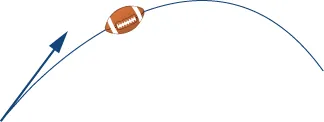46.

A baseball player throws a baseball at an angle of $30°30°$ with the horizontal. If the initial speed of the ball is $100100$ mph, find the horizontal and vertical components of the initial velocity vector of the baseball. (Round to two decimal places.)

47.

A bullet is fired with an initial velocity of $15001500$ ft/sec at an angle of $60°60°$ with the horizontal. Find the horizontal and vertical components of the velocity vector of the bullet. (Round to two decimal places.)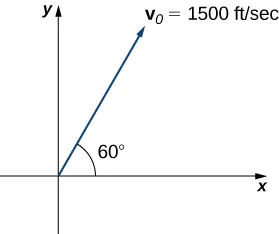48.

[T] A 65-kg sprinter exerts a force of $798798$ N at a $19°19°$ angle with respect to the ground on the starting block at the instant a race begins. Find the horizontal component of the force. (Round to two decimal places.)

49.

[T] Two forces, a horizontal force of $4545$ lb and another of $5252$ lb, act on the same object. The angle between these forces is $25°.25°.$ Find the magnitude and direction angle from the positive x-axis of the resultant force that acts on the object. (Round to two decimal places.)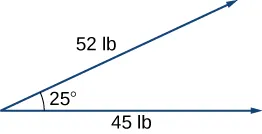50.

[T] Two forces, a vertical force of $2626$ lb and another of $4545$ lb, act on the same object. The angle between these forces is $55°.55°.$ Find the magnitude and direction angle from the positive x-axis of the resultant force that acts on the object. (Round to two decimal places.)

51.

[T] Three forces act on object. Two of the forces have the magnitudes $5858$ N and $2727$ N, and make angles $53°53°$ and $152°,152°,$ respectively, with the positive x-axis. Find the magnitude and the direction angle from the positive x-axis of the third force such that the resultant force acting on the object is zero. (Round to two decimal places.)

52.

Three forces with magnitudes $8080$ lb, $120120$ lb, and $6060$ lb act on an object at angles of $45°,45°,$ $60°60°$ and $30°,30°,$ respectively, with the positive x-axis. Find the magnitude and direction angle from the positive x-axis of the resultant force. (Round to two decimal places.)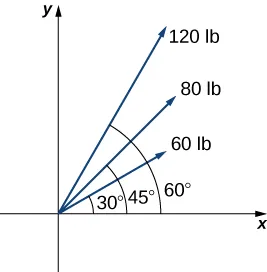53.

[T] An airplane is flying in the direction of $43°43°$ east of north (also abbreviated as $N43E)N43E)$ at a speed of $550550$ mph. A wind with speed $2525$ mph comes from the southwest at a bearing of $N15E.N15E.$ What are the ground speed and new direction of the airplane?54.

[T] A boat is traveling in the water at $3030$ mph in a direction of $N20EN20E$ (that is, $20°20°$ east of north). A strong current is moving at $1515$ mph in a direction of $N45E.N45E.$ What are the new speed and direction of the boat?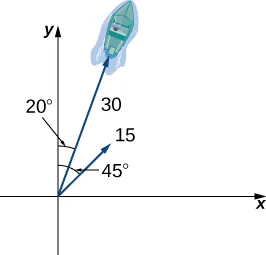55.

[T] A 50-lb weight is hung by a cable so that the two portions of the cable make angles of $40°40°$ and $53°,53°,$ respectively, with the horizontal. Find the magnitudes of the forces of tension $T1T1$ and $T2T2$ in the cables if the resultant force acting on the object is zero. (Round to two decimal places.)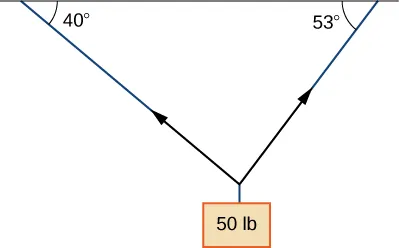56.

[T] A 62-lb weight hangs from a rope that makes the angles of $29°29°$ and $61°,61°,$ respectively, with the horizontal. Find the magnitudes of the forces of tension $T1T1$ and $T2T2$ in the cables if the resultant force acting on the object is zero. (Round to two decimal places.)

57.

[T] A 1500-lb boat is parked on a ramp that makes an angle of $30°30°$ with the horizontal. The boat’s weight vector points downward and is a sum of two vectors: a horizontal vector $v1v1$ that is parallel to the ramp and a vertical vector $v2v2$ that is perpendicular to the inclined surface. The magnitudes of vectors $v1v1$ and $v2v2$ are the horizontal and vertical component, respectively, of the boat’s weight vector. Find the magnitudes of $v1v1$ and $v2.v2.$ (Round to the nearest integer.)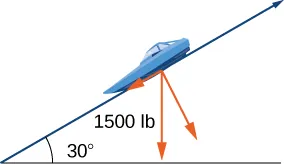58.

[T] An 85-lb box is at rest on a $26°26°$ incline. Determine the magnitude of the force parallel to the incline necessary to keep the box from sliding. (Round to the nearest integer.)

59.

A guy-wire supports a pole that is $7575$ ft high. One end of the wire is attached to the top of the pole and the other end is anchored to the ground $5050$ ft from the base of the pole. Determine the horizontal and vertical components of the force of tension in the wire if its magnitude is $5050$ lb. (Round to the nearest integer.)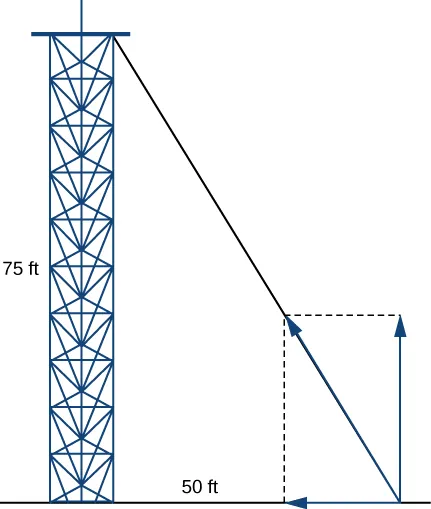60.

A telephone pole guy-wire has an angle of elevation of $35°35°$ with respect to the ground. The force of tension in the guy-wire is $120120$ lb. Find the horizontal and vertical components of the force of tension. (Round to the nearest integer.)

Order a print copy

As an Amazon Associate we earn from qualifying purchases.